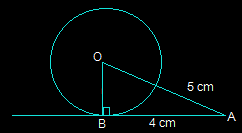Guru

# The length of a tangent from a point A at distance 5 cm from the centre of the circle is 4 cm. Find the radius of the circle. Q.6

• 0

Sir how i solve this question of class 10th of exercise 10.2 of ncert math of question no.6, give me the best and simple way to solve this question The length of a tangent from a point A at distance 5 cm from the centre of the circle is 4 cm. Find the radius of the circle.

Share

1. Draw the diagram as shown below.Here, AB is the tangent that is drawn on the circle from a point A.

So, the radius OB will be perpendicular to AB i.e. OB ⊥ AB

We know, OA = 5cm and AB = 4 cm

Now, In △ABO,

OA2 =AB2+BO2 (Using Pythagoras theorem)

52 = 42+BO2

BO2 = 25-16

BO2 = 9

BO = 3

So, the radius of the given circle i.e. BO is 3 cm.

• 0